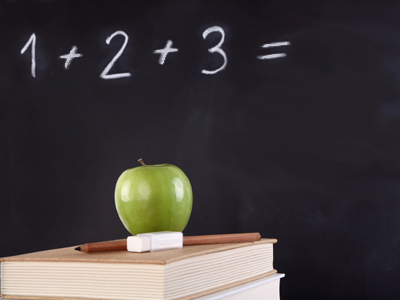1 + 2 + 3 gives the answer 6. So does 3 + 2 + 1. It doesn't matter what order you add the numbers in, you still get the same answer.

# Year 2 Calculation - Addition

This quiz addresses the requirements of the National Curriculum KS1 Maths and Numeracy for children aged 6 and 7 in year 2. Specifically this quiz is aimed at the section dealing with addition of numbers including: a two-digit number and ones, a two-digit number and tens, two two-digit numbers and three one-digit numbers.

Question 1
What is the total of 7 and 26?
34
43
23
33
Starting with the larger number and counting on might be a good plan
Question 2
What is the total of 2, 15 and 3?
20
19
25
15
Additions can be done in any order - putting the 15 first could be useful
Question 3
What is 10 + 27 + 10?
45
37
47
54
Starting with 27 and counting up in tens is a good option to try
Question 4
What is the sum of 27 and 43?
70
80
60
56
Using the number bond of 7 and 3 might be useful
Question 5
What is 8 + 9 + 7?
26
25
23
24
Trying to make 10 and then counting on, or using a near double of 7 + 7 could have helped
Question 6
What is 23 + 45?
75
68
65
57
Partitioning means splitting up the tens and units, making this addition easier
Question 7
What is 30 and 48 added together?
87
78
76
68
Adding the 30 and 40 first and then adding on the units is a good strategy here
Question 8
What is 10 + 9 + 10?
11
39
19
29
Adding the 10 and 10 up first, then adding the 9 might have helped
Question 9
you should always put the largest number first
you should always put the smallest number first
you can add them in any order
Addition calculations can be done in any order, but subtractions cannot
Question 10
What is 3 + 9 + 4?
16
15
21
19